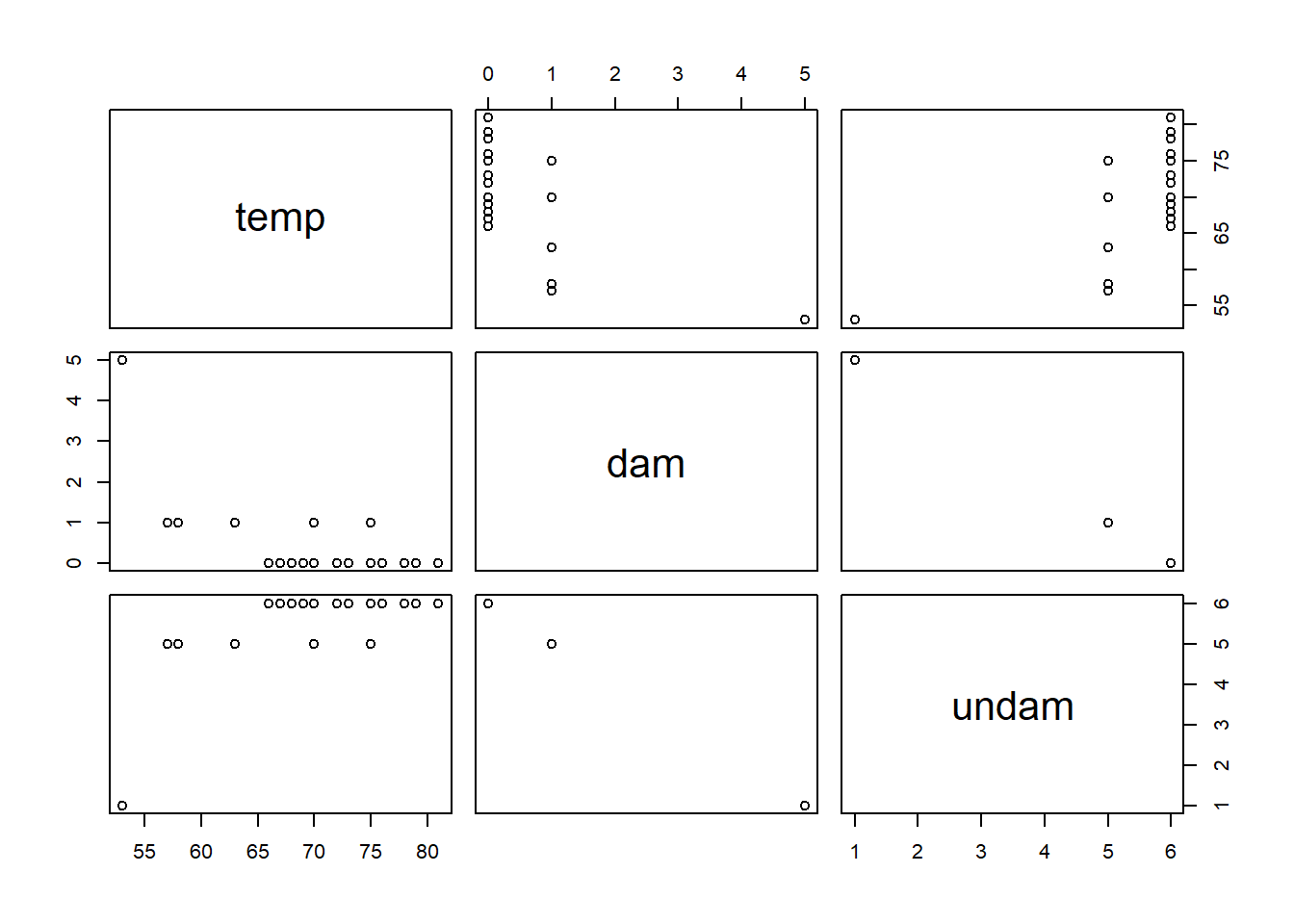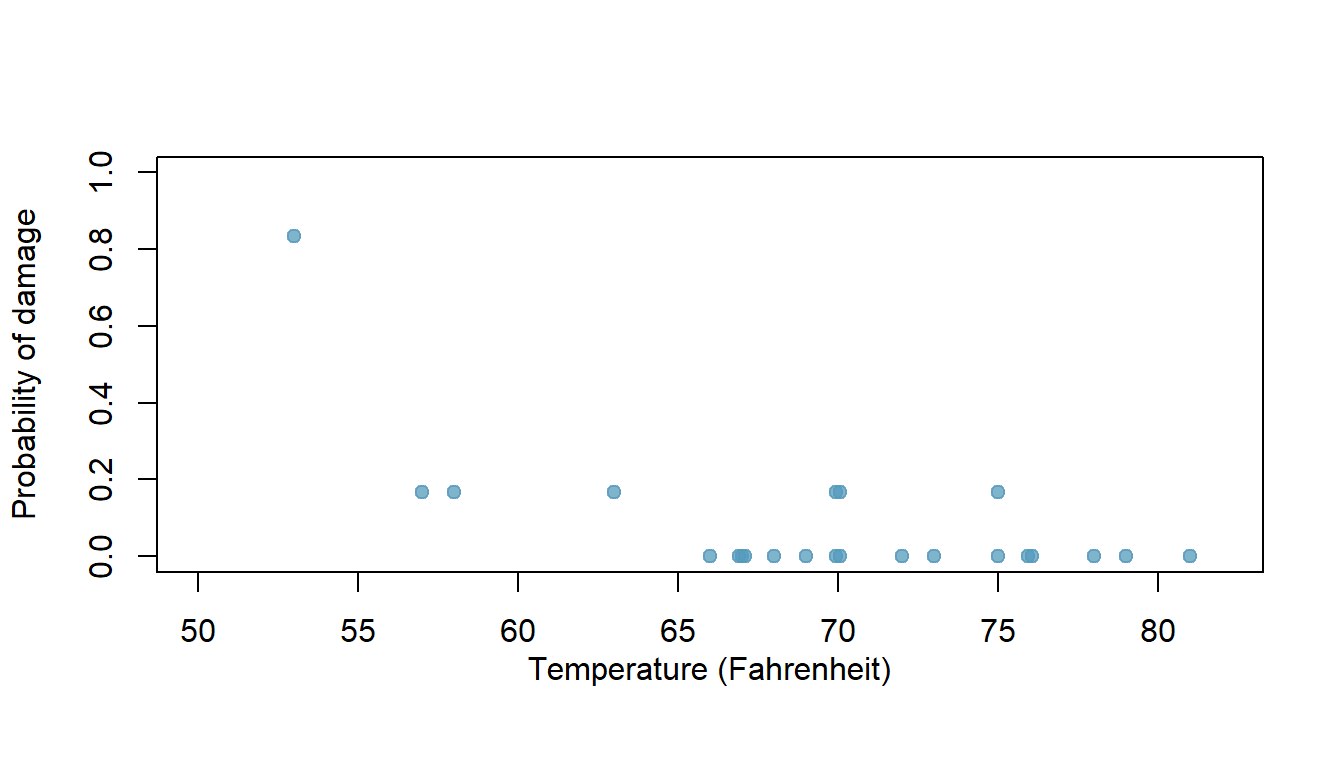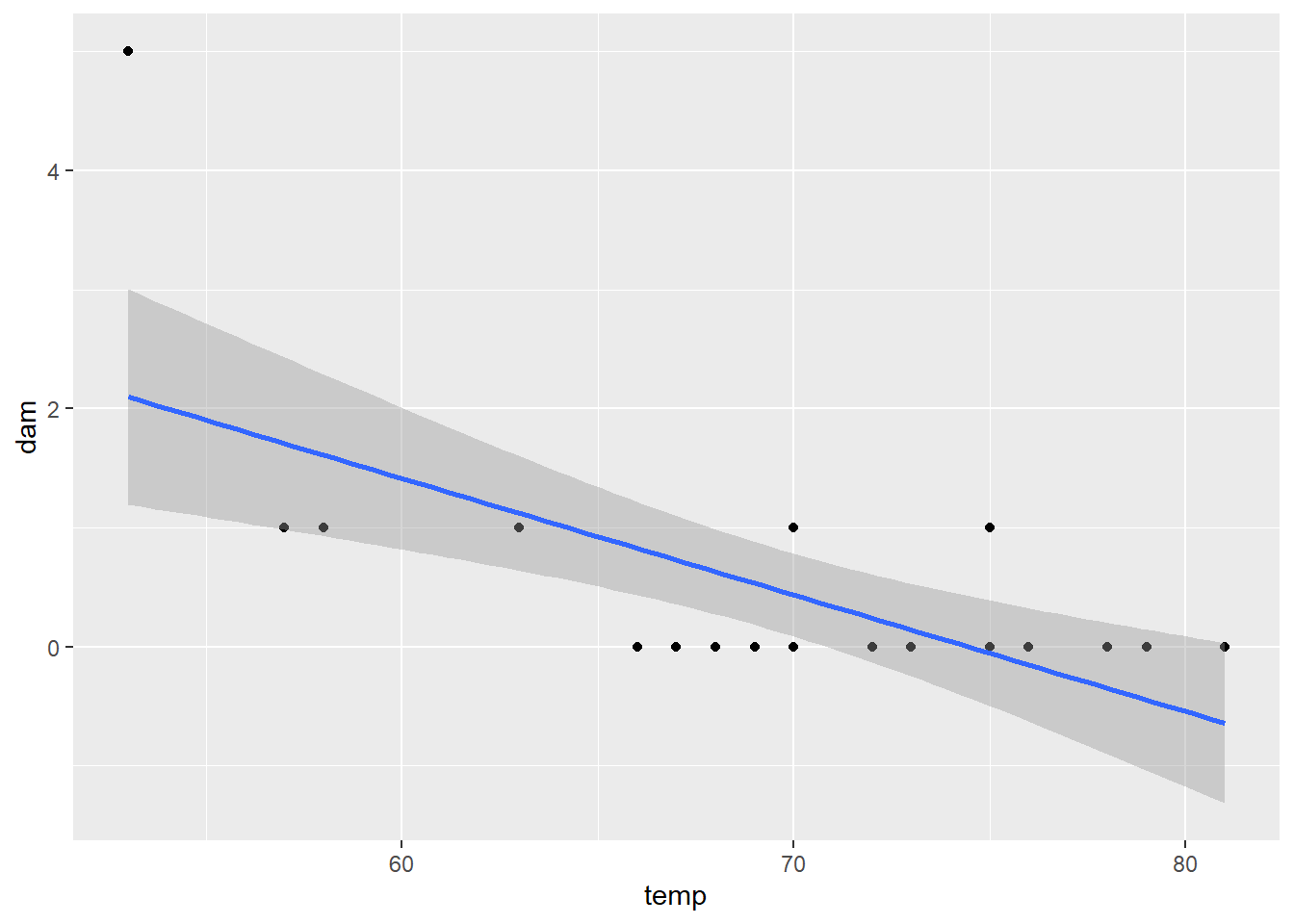Baby weights, Part I. (9.1, p. 350) The Child Health and Development Studies investigate a range of topics. One study considered all pregnancies between 1960 and 1967 among women in the Kaiser Foundation Health Plan in the San Francisco East Bay area. Here, we study the relationship between smoking and weight of the baby. The variable smoke is coded 1 if the mother is a smoker, and 0 if not. The summary table below shows the results of a linear regression model for predicting the average birth weight of babies, measured in ounces, based on the smoking status of the mother.

The variability within the smokers and non-smokers are about equal and the distributions are symmetric. With these conditions satisfied, it is reasonable to apply the model. (Note that we don’t need to check linearity since the predictor has only two levels.)

1. Write the equation of the regression line.

Since we are only dealing with a linear regression model, and the y-intercept = 123.05 and the slope = -8.94 are given, the equation can be written as:

$$\hat{babyweight}$$ = 123.05 −8.94 x smoke

1. Interpret the slope in this context, and calculate the predicted birth weight of babies born to smoker and non-smoker mothers.

The slope indicates the overall estimate for body weight of babies born to mothers who smoke vs. those who do not smoke, like so:

Mother Who Smokes: 123.05 - 8.94 x 1 = 114.11

Mother Who does not smoke: 123.05 - 8.94 x 0 = 123.05

So acording to these simple calculations, The model predicts that babies born to mothers who smoke will weigh 8.94 ounces less than those born to mothers who do not smoke.

1. Is there a statistically significant relationship between the average birth weight and smoking?

H0: β1 = 0 (no difference in variable)

HA: β1 ≠ 0 (there is a difference)

Since the p-value is so small (=0), we would reject the null hypothesis and conclude the slope is not zero. Therefore there is an association (statistically significant) between smoking and birth weights.

Absenteeism, Part I. (9.4, p. 352) Researchers interested in the relationship between absenteeism from school and certain demographic characteristics of children collected data from 146 randomly sampled students in rural New South Wales, Australia, in a particular school year. Below are three observations from this data set.

The summary table below shows the results of a linear regression model for predicting the average number of days absent based on ethnic background (eth: 0 - aboriginal, 1 - not aboriginal), sex (sex: 0 - female, 1 - male), and learner status (lrn: 0 - average learner, 1 - slow learner).

1. Write the equation of the regression line.

$y = \beta_0 + \beta_1 \times eth + \beta_2 \times sex + \beta_3 \times lrn + e$ $y = 18.93 + -9.11 \times eth + 3.10 \times sex + 2.15 \times lrn$

1. Interpret each one of the slopes in this context.

eth: The slope indicates that there is a 9.11 day reduction in the predicted absenteeism when the subject is NO aboriginal.

sex: The slope indicates that there is a 3.10 day increase in the predicted absenteeism when the subject is male.

lrn: The slope indicates that there is a 2.15 day increase in the predicted absenteeism when the subject is a slow learner.

1. Calculate the residual for the first observation in the data set: a student who is aboriginal, male, a slow learner, and missed 2 days of school.
eth <- 0 # Aboriginal
sex <- 1 # Male
lrn <- 1 # Slow Learner
missedActualDays <- 2

predictedDays <- 18.93 - 9.11 * eth + 3.1 * sex + 2.15 * lrn

residual <- missedActualDays - predictedDays
residual
##  -22.18

the residual for the first observation is: -22.18

1. The variance of the residuals is 240.57, and the variance of the number of absent days for all students in the data set is 264.17. Calculate the $$R^2$$ and the adjusted $$R^2$$. Note that there are 146 observations in the data set.
R2 = 1 - (240.57)/(264.17)
R2
##  0.08933641
adjR2 = 1 - (240.57)/(264.17) * ((146-1)/(146-3-1))
adjR2
##  0.07009704

Absenteeism, Part II. (9.8, p. 357) Exercise above considers a model that predicts the number of days absent using three predictors: ethnic background (eth), gender (sex), and learner status (lrn). The table below shows the adjusted R-squared for the model as well as adjusted R-squared values for all models we evaluate in the first step of the backwards elimination process.

Which, if any, variable should be removed from the model first?

Since Adjusted $$R^2$$ = 0.0723, the ‘lrn’ variable should be removed from the model first.

Challenger disaster, Part I. (9.16, p. 380) On January 28, 1986, a routine launch was anticipated for the Challenger space shuttle. Seventy-three seconds into the flight, disaster happened: the shuttle broke apart, killing all seven crew members on board. An investigation into the cause of the disaster focused on a critical seal called an O-ring, and it is believed that damage to these O-rings during a shuttle launch may be related to the ambient temperature during the launch. The table below summarizes observational data on O-rings for 23 shuttle missions, where the mission order is based on the temperature at the time of the launch. Temp gives the temperature in Fahrenheit, Damaged represents the number of damaged O-rings, and Undamaged represents the number of O-rings that were not damaged.

1. Each column of the table above represents a different shuttle mission. Examine these data and describe what you observe with respect to the relationship between temperatures and damaged O-rings.
temp <- c(53,57,58,63,66,67,67,67,68,69,70,70,70,70,72,73,75,75,76,76,78,79,81)
dam <- c(5,1,1,1,0,0,0,0,0,0,1,0,1,0,0,0,0,1,0,0,0,0,0)
undam <- c(1,5,5,5,6,6,6,6,6,6,5,6,5,6,6,6,6,5,6,6,6,6,6)

dfMission <- data.frame(temp, dam, undam)

plot(dfMission)Higher number of damaged O-rings are observed when lower temperatures were recorded

Less number of damaged O-rings are observed when higher temperatures were recorded

1. Failures have been coded as 1 for a damaged O-ring and 0 for an undamaged O-ring, and a logistic regression model was fit to these data. A summary of this model is given below. Describe the key components of this summary table in words.

Two components here, the intercept and the temperature. Estimates tells us the model’s parametere estimate. the Z and the p values tell us how good the varibles predict the model.

1. Write out the logistic model using the point estimates of the model parameters.

$log_e\binom{p_i}{1-p_i} = 11.6630 − 0.2162 \times Temperature$

1. Based on the model, do you think concerns regarding O-rings are justified? Explain.

We can see that based on this data, there is a high probability of temperature having effect on the O-rings. The concerns are justified because of the p value being 0.

Challenger disaster, Part II. (9.18, p. 381) Exercise above introduced us to O-rings that were identified as a plausible explanation for the breakup of the Challenger space shuttle 73 seconds into takeoff in 1986. The investigation found that the ambient temperature at the time of the shuttle launch was closely related to the damage of O-rings, which are a critical component of the shuttle. See this earlier exercise if you would like to browse the original data.

\begin{center}\end{center}

1. The data provided in the previous exercise are shown in the plot. The logistic model fit to these data may be written as \begin{align*} \log\left( \frac{\hat{p}}{1 - \hat{p}} \right) = 11.6630 - 0.2162\times Temperature \end{align*}

where $$\hat{p}$$ is the model-estimated probability that an O-ring will become damaged. Use the model to calculate the probability that an O-ring will become damaged at each of the following ambient temperatures: 51, 53, and 55 degrees Fahrenheit. The model-estimated probabilities for several additional ambient temperatures are provided below, where subscripts indicate the temperature:

\begin{align*} &\hat{p}_{57} = 0.341 && \hat{p}_{59} = 0.251 && \hat{p}_{61} = 0.179 && \hat{p}_{63} = 0.124 \\ &\hat{p}_{65} = 0.084 && \hat{p}_{67} = 0.056 && \hat{p}_{69} = 0.037 && \hat{p}_{71} = 0.024 \end{align*}

We have: $log_e\binom{\hat{p}}{1-\hat{p}} = 11.6630 − 0.2162 \times Temperature$

solving for p, we get: $\hat{p} = e^{11.6630 − 0.2162 \times Temperature}/{1+e^{11.6630 − 0.2162 \times Temperature}}$

p51 <- exp((11.663 - 0.2162*51))/(1+exp((11.663 - 0.2162*51)))
p51
##  0.6540297
p53 <- exp((11.663 - 0.2162*53))/(1+exp((11.663 - 0.2162*53)))
p53
##  0.5509228
p55 <- exp((11.663 - 0.2162*55))/(1+exp((11.663 - 0.2162*55)))
p55
##  0.4432456
1. Add the model-estimated probabilities from part~(a) on the plot, then connect these dots using a smooth curve to represent the model-estimated probabilities.
library(ggplot2)
##
## Attaching package: 'ggplot2'
## The following object is masked from 'package:openintro':
##
##     diamonds
ggplot(dfMission,aes(x=temp,y=dam)) + geom_point() +
stat_smooth(method = 'glm', family = 'binomial')
## Warning: Ignoring unknown parameters: family1. Describe any concerns you may have regarding applying logistic regression in this application, and note any assumptions that are required to accept the model’s validity.

Condition for Logistic Regression:

IN order to fit a logistic regression model, we need the following conditions:

- Each predictor xi is linearly related to logit(pi) if all other predictors areheld constant.

- Each outcome Yi is independent of the other outcomes.

The 2 conditions are definitly met.